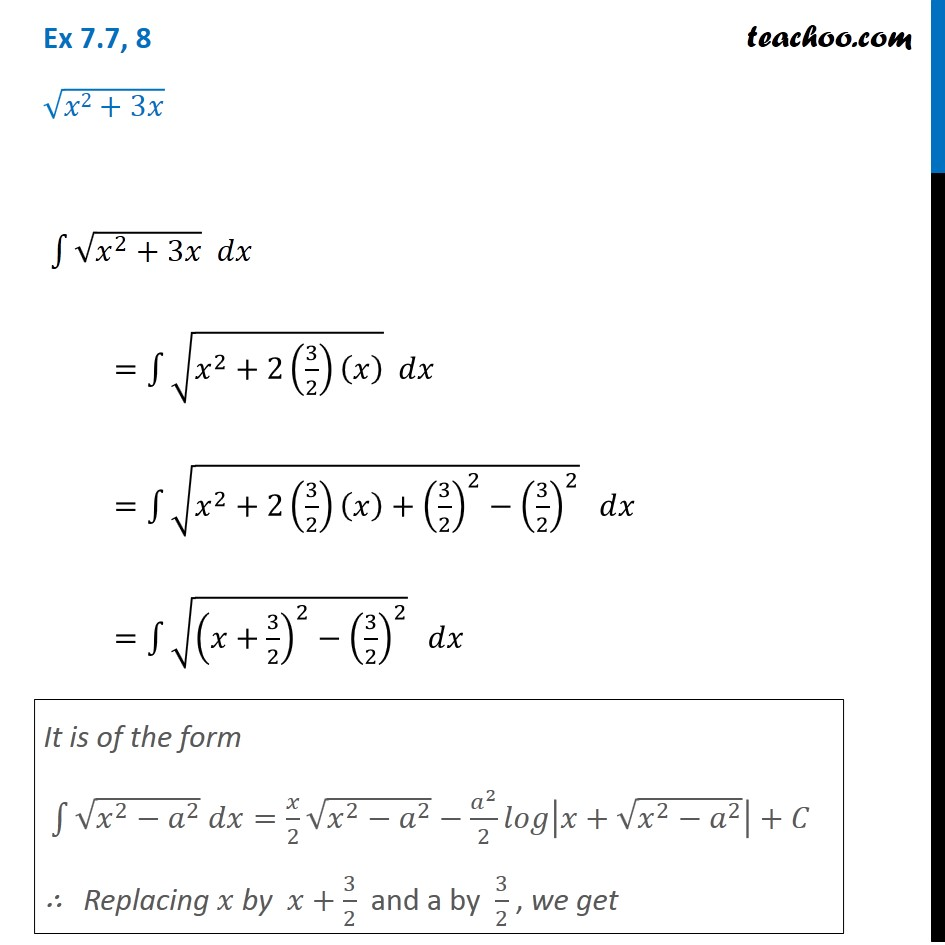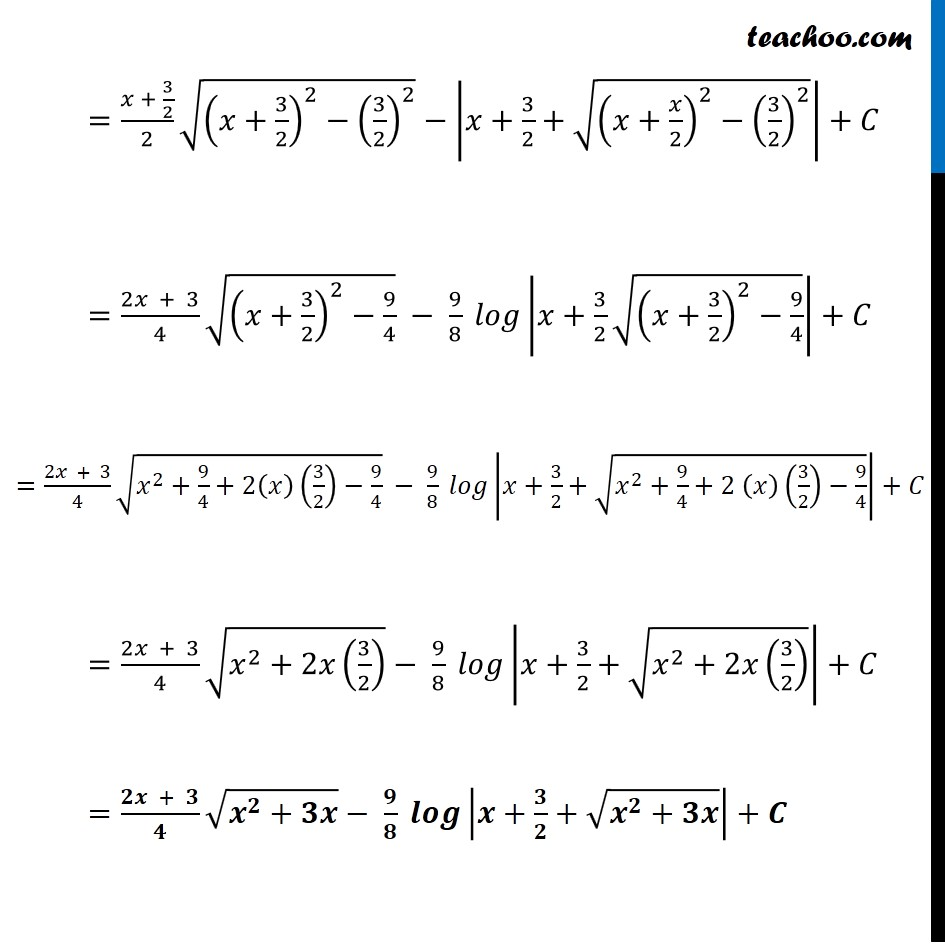Ex 7.7

Chapter 7 Class 12 Integrals
Serial order wiseGet live Maths 1-on-1 Classs - Class 6 to 12

### Transcript

Ex 7.7, 8 √(𝑥2+3𝑥) ∫1▒〖√(𝑥^2+3𝑥) 𝑑𝑥〗 =∫1▒〖√(𝑥^2+2(3/2)(𝑥) ) 𝑑𝑥〗 =∫1▒〖√(𝑥^2+2(3/2)(𝑥)+(3/2)^2−(3/2)^2 ) 𝑑𝑥〗 =∫1▒〖√((𝑥+3/2)^2−(3/2)^2 ) 𝑑𝑥〗 It is of the form ∫1▒〖√(𝑥^2−𝑎^2 ) 𝑑𝑥=𝑥/2 √(𝑥^2−𝑎^2 )−𝑎^2/2 𝑙𝑜𝑔|𝑥+√(𝑥^2−𝑎^2 )|+𝐶〗 ∴ Replacing 𝑥 by 𝑥+3/2 and a by 3/2 , we get =(𝑥 + 3/2)/2 √((𝑥+3/2)^2−(3/2)^2 )−|𝑥+3/2+√((𝑥+𝑥/2)^2−(3/2)^2 )|+𝐶 =(2𝑥 + 3)/4 √((𝑥+3/2)^2−9/4) − 9/8 𝑙𝑜𝑔|𝑥+3/2 √((𝑥+3/2)^2−9/4)|+𝐶 =(2𝑥 + 3)/4 √(𝑥^2+9/4+2(𝑥)(3/2)−9/4) − 9/8 𝑙𝑜𝑔|𝑥+3/2+√(𝑥^2+9/4+2 (𝑥)(3/2)−9/4)|+𝐶 =(2𝑥 + 3)/4 √(𝑥^2+2𝑥(3/2) )− 9/8 𝑙𝑜𝑔|𝑥+3/2+√(𝑥^2+2𝑥(3/2) )|+𝐶 =(𝟐𝒙 + 𝟑)/𝟒 √(𝒙^𝟐+𝟑𝒙)− 𝟗/𝟖 𝒍𝒐𝒈|𝒙+𝟑/𝟐+√(𝒙^𝟐+𝟑𝒙)|+𝑪